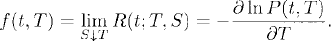# INSTANTANEOUS FORWARD RATE MODELS

The short rate models presented in the previous chapter are not flexible for calibration. As a consequence, Heath et al. (1992) introduced a new uniform framework, called the HJM framework, for modeling the interest rates. In the Heath-Jarrow-Morton (HJM) framework, the entire forward curve is modeled directly. These interest rate models are called HJM models or instantaneous forward rate models. In this chapter, we introduce some concepts related to the HJM framework.

# 46.1 Basic Concepts and Facts

Definition 46.1 (Forward Rate). Let 0 ≤ t < T < S. The simple (or simply compounded) forward rate for [T, S] prevailing at t is defined to bewhere P(t, T) is the value at time t of a dollar at time T. The continuously compounded forward rate for [T, S] prevailing at t is defined to beThe instantaneous forward rate with maturity T prevailing at t is defined asThe function Tf(t, T) is called the forward curve at time t.

Definition 46.2 (Forward Contract). Let t < T. A forward contract on an underlying, entered at time t, with maturity T is ...

Get Measure, Probability, and Mathematical Finance: A Problem-Oriented Approach now with the O’Reilly learning platform.

O’Reilly members experience live online training, plus books, videos, and digital content from nearly 200 publishers.